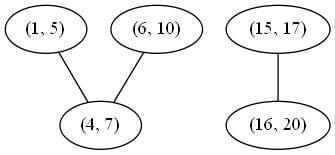Reference: LeetCode
Difficulty: Medium

## Problem

Given a collection of intervals, merge all overlapping intervals.

Note:

Example:

## Analysis

### Brute-Force

From LeetCode solution section:The basic idea is to reduce the original problem to a graph problem. Since [1, 5], [4, 7], and [6, 10] are overlapping, we construct a connected component of them. After we consider all other intervals, we can have a new interval corresponding to each connected component.

Time: $O(N^2) = O(V + E) = O(V) + O(E) = O(N) + O(N^2)$
Space: $O(N^2)$ when all intervals are mutually overlapping.

### Sorting

The idea is to sort all intervals by starting time. After sorting, we have the following cases to handle

Here is my original code:

I don’t why I made it difficult. We can just set the first interval as prev rather than set it as Integer.MIN_VALUE.

Time: $O(N\log{N})$
Space: $O(N)$

CommentJunhao Wang
Hi, I was a master student at USC studying Computer Science.